Rakuten Marketing UKHi, what are you looking for?

# The Ultimate Guide To Excel Formulas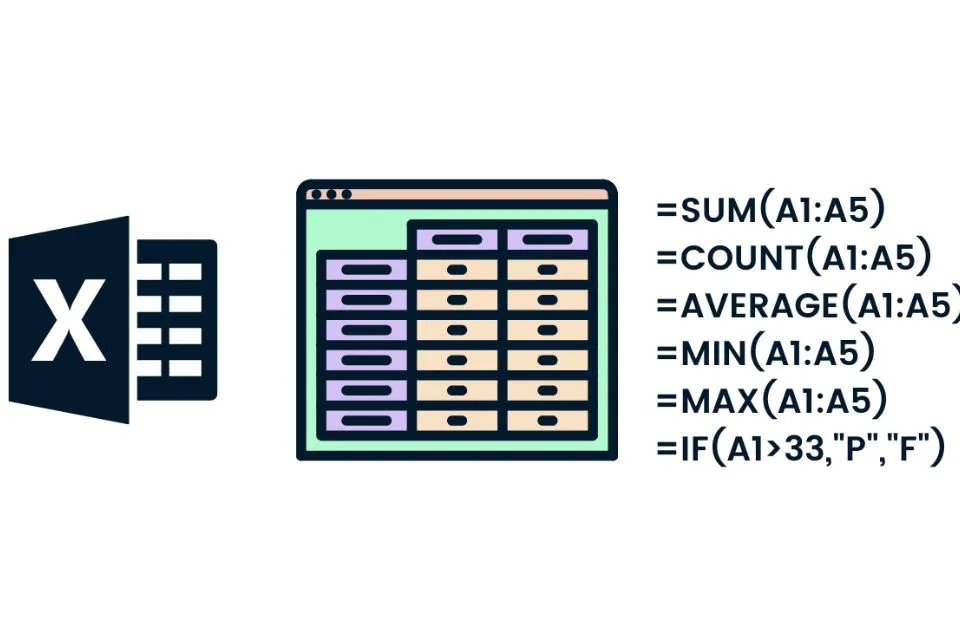excel formulas | hermagic

Excel formulas are the foundation of the Excel spreadsheet application. It is the language used to create and manipulate data within a workbook. It is a powerful tool that allows users to quickly manipulate large amounts of data and produce detailed reports and graphics. Excel formulas provide a range of capabilities to make data analysis easier and faster.

Excel formulas are simple and easy to understand. Most of the time they are self-explanatory, although there is an extensive library of online help available if needed. Excel formulas are made up of functions, operators, and values. Functions are used to perform calculations on the data, such as adding, subtracting, multiplying, and dividing.

Formulas can be entered manually into a cell or can be selected from a library of predefined formulas. These predefined formulas are very powerful and can make many calculations with a single formula. For example, the SUM function calculates the sum of a range of cells, while the AVERAGE function calculates the average of a range of cells.

These formulas can be combined to create even more complex calculations. Formulas can also be used to automate calculations. For example, a formula can be used to calculate the total sales for a particular month.

This can be done by combining the SUM function with the IF function, which allows the user to specify a condition that must be met in order for the formula to be applied. This can save a lot of time and effort, as the user does not need to manually calculate the sales for each month.

## Features of Excel formulas

Excel formulas are an essential part of spreadsheet software. They allow users to quickly and easily perform complex calculations and data analysis. Excel formulas can also be used to automate repetitive tasks, such as creating charts and graphs and can even be used to create custom functions. In this essay, we will discuss the features of Excel formulas and their applications.

### 1. Manipulation of Data

Excel formulas can be used to manipulate data in various ways. For example, they can be used to add, subtract, multiply, and divide numbers, as well as to compare numbers and strings. Excel formulas can also be used to calculate the average, maximum, and minimum values of a range of data. They can also be used to filter, sort, and group data.

### 2. Logical Functions

Excel formulas can also be used to evaluate logical conditions. These functions allow users to determine if a certain value is true or false. This can be used to create conditional formatting rules, which can be used to highlight specific data or to apply font or background colors to cells.

### 3. Lookup and Reference Functions

Excel formulas can also be used to look up specific data in a table or range of cells. For example, the VLOOKUP function can be used to look up a specific value in a table and return a result from a different column. This can be used to quickly and easily search for information in large datasets.

### 4. Text Functions

Excel formulas can also be used to manipulate text data. These functions can be used to extract, combine, and format text strings. For example, the LEFT and RIGHT functions can be used to extract a specific number of characters from the beginning or end of a string.

### 5. Date and Time Functions

Excel formulas can also be used to calculate date and time values. These functions can be used to add or subtract a certain number of days from a date, or to calculate the difference between two dates. They can also be used to calculate the current date and time or to convert a date into its corresponding day of the week.

### 6. Percentage formula

The Excel percentage formula is used to calculate a percentage of a number. This formula can be used to determine a percentage of a total or to determine the percentage of a number relative to another number. To calculate a percentage in Excel, the user must first type in the numerator and denominator of the fraction.

## Conclusion

Excel formulas are a powerful tool for manipulating and analyzing data. They enable users to automate calculations, create custom formulas and perform calculations with ease. With a few simple steps, Excel formulas can help you quickly and accurately analyze and summarize your data. With the wide range of functions available, Excel formulas open up a world of possibilities for data analysis. For more information visit Datacamp and the official website of Hermagic.

## FAQ’s

What are the 7 basic Excel formulas?
SUM: This formula adds the values in a range of cells.

1. AVERAGE: This formula averages the numbers in a range of cells.
2. COUNT: This formula counts the number of cells that contain numbers within a range.
3. MAX: This formula finds the maximum value in a range of cells.
4. MIN: This formula finds the minimum value in a range of cells.
5. IF: This formula checks whether a condition is true or false and returns a value based on the result.
6. VLOOKUP: This formula looks up a value in the leftmost column of a table and returns a value in the same row from a column you specify.
What are the top 10 Excel formulas?
SUM: To add up a range or multiple numbers
1. AVERAGE: To calculate the average of a range of values
2. COUNT: To count the number of cells with numerical values
3. IF: To execute logical tests and return different values
4. VLOOKUP: To search for a value in a table and return a corresponding value
5. INDEX: To return a value from a list or table of data
6. CONCATENATE: To join two or more strings together
7. MATCH: To search for a value in a range of values and return its relative position
8. MAX/MIN: To find the largest/smallest value in a range
9. ROUND: To round a number to a specified number of digits
What are the 20 functions of Excel?
• Basic data sorting and filtering
• Sum and average calculations
• Lookup and reference functions
• Conditional formatting
• Text functions
• Financial functions
• Date and time functions
• Statistical functions
• Database functions
• Array formulas
• Goal seek
• Scenario manager
• Data validation
• What-if analysis
• Text-to-columns
• Data consolidation
• Pivot tables and charts
• Web queries
• Solver
• Macro recording and automation
• ## Disclaimer: Affiliate policy - I may earn a commission when you buy through the links available in the above blog.

### Fashion & Beauty

For a long time, women have had to wear uncomfortable clothing to fit in with societal norms. It is difficult to find clothing that...

### Fashion

Ah, college. Chilling with friends, staying up all night working on assignments, and making life memories, Farfetch Studentare rites of passage for a fulfilling...

### Health & Wellness

What are Rice Water’s Hair Benefits? With so many passing fads on TikTok, it’s easy to dismiss the use of rice water in the...

### Accessories

Smooth glowing skin can be your best feature, but there are a million things stopping us from flaunting our perfect skin and messing with...ENEN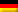DE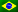PT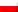PL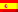ES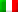IT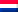NL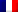FR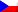CS
You Will Absolutely Love These Designer Clothing For Men In 2022 Workout In Style With The Top Nike Headbands In 2022 What Is A Wetsuit, And What Is Its Purpose? Wear These Slips & Control Pants For Tummy For A Perfect Fit Of Your Outfit Wear These Best Chino Pants For Men For A Formal Look
You Will Absolutely Love These Designer Clothing For Men In 2022 Workout In Style With The Top Nike Headbands In 2022 What Is A Wetsuit, And What Is Its Purpose? Wear These Slips & Control Pants For Tummy For A Perfect Fit Of Your Outfit Wear These Best Chino Pants For Men For A Formal Look